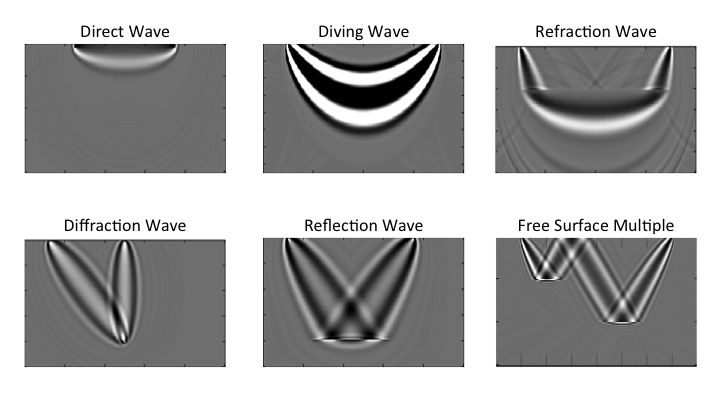# CSIM Wave-equation Series Lab

By Xin Wang (xin.wang@kaust.edu.sa), Bldg 1, 3139-WS13, 808-0386

### PART 4: Wavepath labCSIM Wave equation Series Lab Part 1: TDFD solver to acoustic wave equation lab PART 2: Reverse time migration lab PART 3: Born modeling and adjoint test lab PART 4: Wavepath lab Objective: Learn and discuss the wave path of different type of waves. Theory: For direct, diving and refraction wave, just use the conventional rtm code and mute other events but only themselves left: WP   =   P   •   Q P is the forward propagation field, and Q is the backward propagation field. For reflection and diffraction wave, a reflectivity model and an additional born modeling is needed: WP   =   P   •   PP    +   Q   •   QQ PP is the backward pertubation field, which is calculated by a born modeling of the pertubated reflectivity and the background wave field Q; and QQ the forward pertubation field, which is calculated by a born modeling of the pertubated reflectivity and the background wave field P. Procedure: Direct wave wavepath Define a homogenerous velocity model, and the model parameters; nz=81;nx=201;vel=zeros(nz,nx)+1000; dx=5; x = (0:nx-1)*dx; z = (0:nz-1)*dx; Define the source and receiver geometry; sx = (nx-1)/4*dx; sz = 0; gx=(0:2:(nx-1))*dx; gz=zeros(size(gx)); g=1:numel(gx); Setup FD parameters and source wavelet; nbc=40; nt=2001; dt=0.0005; t=(0:nt-1)*dt;isFS=false;freq=25; s=ricker(freq,dt); Generate the synthetic data; tic; seis=a2d_mod_abc28(vel,nbc,dx,nt,dt,s,sx,sz,gx,gz,isFS);toc; Calculate and plot the wavepath for direct wave; figure(1);set(gcf,'position',[0 0 1000 500]); subplot(221);imagesc(x,z,vel);colorbar;xlabel('X (m)'); ylabel('Z (m)'); title('velocity'); figure(1);subplot(222);imagesc(g,t,seis);colormap(gray); xlabel('Time (t)'); title('seismic gather'); figure(1);subplot(223);plot(t,seis(:,76));xlabel('Time (t)'); title('seismic trace'); tic; wp=a2d_wavepath_abc28(seis(:,76),vel,nbc,dx,nt,dt,s,sx,sz,gx(76),gz(76)); toc; figure(1);subplot(224);imagesc(x,z,wp);colormap(gray);caxis([-10 10]); xlabel('X (m)'); ylabel('Z (m)'); title('wave path for direct wave'); Diving wave wavepath Define a gradient velocity model, and the model parameters; vel=(1000:1000/150:2000);vel=repmat(vel',[1,401]); [nz,nx]=size(vel);x = (0:nx-1)*dx; z = (0:nz-1)*dx; Define the source and receiver geometry; sx = (nx-1)/8*dx; sz = 0; gx=(0:2:nx-1)*dx; gz=zeros(size(gx)); g=0:numel(gx); Setup FD parameters and source wavelet; nbc=40; nt=4001; dt=0.0005; t=(0:nt-1)*dt;isFS=false;freq=25; s=ricker(freq,dt); Generate the synthetic data; tic; seis=a2d_mod_abc28(vel,nbc,dx,nt,dt,s,sx,sz,gx,gz,isFS);toc; Calculate and plot the wavepath for diving wave; figure(2);set(gcf,'position',[0 0 1000 500]); subplot(221);imagesc(x,z,vel);colorbar; xlabel('X (m)'); ylabel('Z (m)'); title('velocity'); figure(2);subplot(222);imagesc(g,t,seis);colormap(gray);caxis([-1 1]); xlabel('Time (t)'); title('seismic gather'); gx=gx(176);gz=gz(176); trace=seis(:,176); trace(2901:end)=0; figure(2);subplot(223);plot(t,trace); xlabel('Time (t)'); title('seismic trace with direct wave muted'); tic; wp=a2d_wavepath_abc28(trace,vel,nbc,dx,nt,dt,s,sx,sz,gx,gz); toc; figure(2);subplot(224);imagesc(x,z,wp);colormap(gray);caxis([-0.1 0.1]); xlabel('X (m)'); ylabel('Z (m)'); title('wave path for diving wave'); Refraction wave wavepath Define a two layer velocity model, and the model parameters; vel=[repmat(1000,[1 41]),repmat(2000,[1,80])];vel=repmat(vel',[1,401]); [nz,nx]=size(vel);x = (0:nx-1)*dx; z = (0:nz-1)*dx; Define the source and receiver geometry; sx = (nx-1)/8*dx; sz = 0; gx=(0:2:nx-1)*dx; gz=zeros(size(gx)); g=0:numel(gx); Setup FD parameters and source wavelet; nbc=80; nt=4001; dt=0.0005; t=(0:nt-1)*dt;isFS=false;freq=25; s=ricker(freq,dt); Generate the synthetic data, and mute events other than refraction. tic; seis=a2d_mod_abc28(vel,nbc,dx,nt,dt,s,sx,sz,gx,gz,isFS);toc; trace=seis(:,176); trace(2801:end)=0; Calculate and plot the wavepath for refraction wave; figure(3);set(gcf,'position',[0 0 1000 500]); subplot(221);imagesc(x,z,vel);colorbar;xlabel('X (m)'); ylabel('Z (m)'); title('velocity'); figure(3);subplot(222);imagesc(g,t,seis);colormap(gray);caxis([-0.025 0.025]);xlabel('Time (t)'); title('seismic data'); figure(3);subplot(223);plot(t,trace);xlabel('Time (t)'); title('refraction wave'); tic; wp=a2d_wavepath_abc28(trace,vel,nbc,dx,nt,dt,s,sx,sz,gx(176),gz(176)); toc; figure(3);subplot(224);imagesc(x,z,wp);colormap(gray);caxis([-0.0002 0.0002]); xlabel('X (m)'); ylabel('Z (m)'); title('wave path for refraction wave'); Diffraction wave wavepath Define a point scatterrer model, and the model parameters; vel=zeros(101,201)+1000; vel(81,101)=1200;[nz,nx]=size(vel);x = (0:nx-1)*dx; z = (0:nz-1)*dx; Define the source and receiver geometry; sx = (nx-1)/8*dx; sz = 0; gx=(0:2:nx-1)*dx; gz=zeros(size(gx)); g=0:numel(gx); Setup FD parameters and source wavelet; nbc=80; nt=2601; dt=0.0005; t=(0:nt-1)*dt;isFS=false;freq=20; s=ricker(freq,dt); Smooth the background velocity; [vel_ss,refl_ss]=vel_smooth(vel,3,3,1); Generate the synthetic data, and mute events other than diffraction. tic; seis=a2d_mod_abc28(vel,nbc,dx,nt,dt,s,sx,sz,gx,gz,isFS);toc; trace=seis(:,51); trace(1:1800)=0;trace(2301:end)=0; Calculate and plot the wavepath for diffraction wave; figure(4);set(gcf,'position',[0 0 1000 500]); subplot(221);imagesc(x,z,vel);colorbar;xlabel('X (m)'); ylabel('Z (m)'); title('velocity'); figure(4);subplot(222);imagesc(g,t,seis(1:2601,:));colormap(gray);caxis([-0.025 0.025]); xlabel('Time (t)'); title('seismic data for one trace'); figure(4);subplot(223);plot(t,trace);xlabel('Time (t)'); title('diffraction wave'); tic; wp=a2d_wavepath_abc28_refl(trace,vel_ss,refl_ss,nbc,dx,nt,dt,s,sx,sz,gx(51),gz(51)); toc; figure(4);subplot(224);imagesc(x,z,wp);colormap(gray);caxis([-0.002 0.002]); xlabel('X (m)'); ylabel('Z (m)'); title('wave path for diffraction wave'); Reflection wave wavepath Define a two layer model, and the model parameters; vel=zeros(101,201)+1000; vel(81:end,:)=1500;[nz,nx]=size(vel);x = (0:nx-1)*dx; z = (0:nz-1)*dx; Define the source and receiver geometry; sx = (nx-1)/8*dx; sz = 0; gx=(0:2:nx-1)*dx; gz=zeros(size(gx)); g=0:numel(gx); Setup FD parameters and source wavelet; nbc=80; nt=2601; dt=0.0005; t=(0:nt-1)*dt;isFS=false;freq=20; s=ricker(freq,dt); Smooth the background velocity; [vel_ss,refl_ss]=vel_smooth(vel,3,3,1); Generate the synthetic data, and mute events other than diffraction. tic; seis=a2d_mod_abc28(vel,nbc,dx,nt,dt,s,sx,sz,gx,gz,isFS);toc; trace=seis(:,76); trace(1:1800)=0;trace(2301:end)=0; Calculate and plot the wavepath for reflection wave; figure(5);set(gcf,'position',[0 0 1000 500]); subplot(221);imagesc(x,z,vel);colorbar; xlabel('X (m)'); ylabel('Z (m)'); title('velocity'); figure(5);subplot(222);imagesc(g,t,seis(1:2601,:));colormap(gray);caxis([-0.1 0.1]); xlabel('Time (t)'); title('seismic data for one trace'); figure(5);subplot(223);plot(t,trace);xlabel('Time (t)'); title('reflection wave'); tic; wp=a2d_wavepath_abc28_refl(trace,vel,refl_ss,nbc,dx,nt,dt,s,sx,sz,gx(76),gz(76)); toc; figure(5);subplot(224);imagesc(x,z,wp);colormap(gray);caxis([-2 2]); xlabel('X (m)'); ylabel('Z (m)'); title('wave path for reflection wave'); Reminder: For all labs, you can copy the bold line command to a single script, and run the scripts to generate the same results. References: Seismic Inversion, Gerard T. Schuster